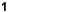# CYDONIAN MOUND GEOMETRY:

## A Closer Look

### Abstract

` `
In Dr. Horace W. Crater's analysis of the mound configuration located in the Cydonia area of Mars It was pointed out that a group of five small, relatively distinct and uniform features referred to as mounds labeled GABDE correspond to the positions of five nodes (corners and side midpoints) of a classic geometric figure known as a "square root 2 (sqrt2) rectangle.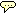The present paper presents a detailed account of the geometric relationships between the mound pentad, the geometry of the tetrahedron, and the geometry of the sqrt2 rectangle. A consideration of possible cultural or symbolic meaning for this geometric formation is also presented.

### 1. Introduction

Is the investigation of geometric relationships between objects on another planet in order to evaluate their possible artificiality a legitimate endeavor? one scientist has stated that since geologists would never consider making such "planimetric measurements," there is no point in anyone else doing so. But when questions of possible cultural or symbolic meaning arise, geology is not the relevant science. Rather it is those disciplines which study systems of cultural symbolism and other manifestations of culture -- archaeologists, anthropologists, and experts in the communication of ideas by means of symbols. Geometry provides a universal "language" for the expression of both aesthetic and symbolic ideas. There is no clear reason why that potential universality should not apply to extraterrestrial intelligence as well as Earthly cultures. In searching for a possible meaning of the mound configuration at Cydonia, it is relevant and appropriate to explore the geometric and possible symbolic characteristics of the mound configuration.` `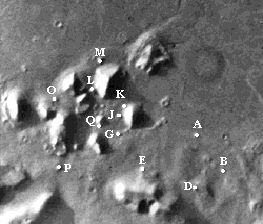##### Cydonia: Mound Letter Designations

Questions that bear on the issue of artificiality are the following:

1.   Does the mound geometry conform to some understandable grid?
2.   Is the mound geometry commonplace or unique?
3.   Is the mound geometry unproductive or rich?
4.   Is there any recognizable cultural or symbolic quality in the patterns
that might provide a clue to possible meaning or utility?

In the discussion below, we will try to answer these questions.

` `

### 2. The Tetrahedron Cross-section

The two types of triangles that were found to generate a radical statistical anomaly among the 12 mounds at Cydonia are those containing angles related to the cross-section of a tetrahedron. We begin with a diagram showing how the cross-section, which is an isosceles triangle, appears within the tetrahedron.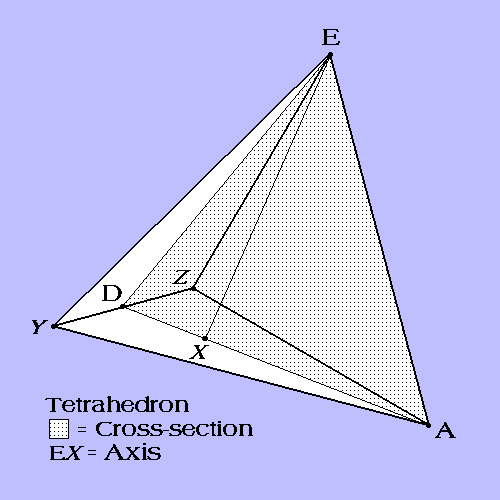Diagram 1

Mounds EAD mark out a triangle matching the cross-section (shaded area) of tetrahedron EAYZ.

In this diagram, line EA is one edge of the tetrahedron with point E at the apex. Point D lies at the midpoint of the opposite edge, and line AD bisects the base of the tetrahedron. Line EX represents the axis of the tetrahedron. Point X is equidistant from A, Y, and Z on the base of the tetrahedron. Line EX is also an altitude of the cross-section as well as the axis of the tetrahedron. (Altitude = line from any vertex drawn perpendicular to the opposite side.)

If we draw all the altitudes of the cross-section we find that it is divided into triangles having certain characteristic geometric properties. Let us look first at the two triangles formed within the cross-section by altitude EX.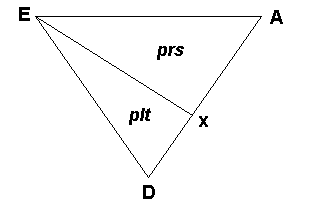Diagram 2

Altitude EX divides the cross section into prs and plt triangles.

This basic division, using the axis of the implied tetrahedron, divides the cross-section into triangles we have termed "prs" and "plt" triangles with the following internal angles to be designated p, t, l, r, and s (see diagrams 5a,b and 6a,b below for the exact location of each of these angles within the triangles):

```	Abbreviation	Degrees		Radians
----------------------------------------------------------------
p		90		pi/2
t		19.5		0.34
l		70.5		(p - t)
r		54.75		(p + t) / 2
s		35.25		(p - t ) / 2
```

All the angles are simple functions of pi and another angle t = 19.5 degrees (0.34 radians), which turns out to be a tetrahedral constant, as will be explained below.These triangles have specific roles to play in this particular geometric form. Let's take a look at what happens when you divide the cross-section into subtriangles by drawing all three altitudes.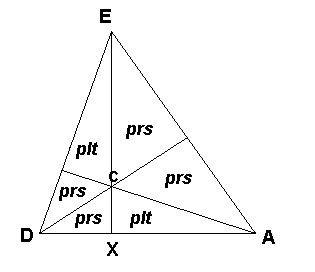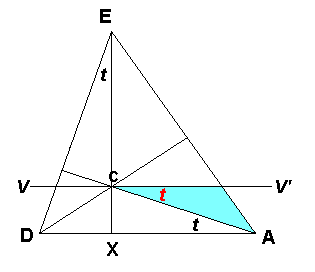Diagrams 3a (left) and 3b (right)

Division of the cross-section by all its altitudes, and the geometric result of a line drawn through the center parallel to the base.

When we divide the cross-section by all three altitudes, multiple instances of prs and plt triangles spring into being, the precise geometric center of the tetrahedron (at C) is identified, and one specific angle -- the tetrahedral constant "t" -- takes on an important role. Because the angle formed by the altitude at A equals t, line V-V' drawn through the center point parallel to AD forms another instance of the angle t (shown in red). Below we will explain the geometric consequences of this fact.

### 3. The Tetrahedral Latitude

The presence of angle t at V'CA is of considerable geometric significance. It determines the value known as the "tetrahedral latitude" (19.5 degrees). This is the latitude where the base of a tetrahedron, when embedded within a sphere, meets the surface of the sphere (taking the apex of the tetrahedron as a pole of the sphere). Diagram 4 below graphically demonstrates how this comes about.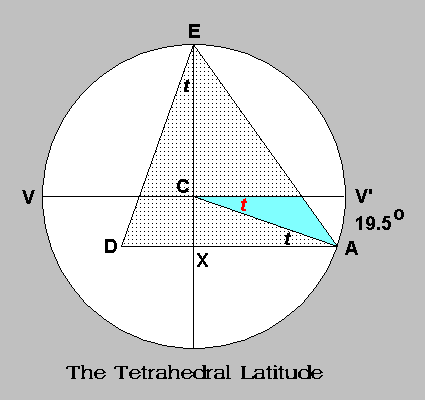Diagram 4

Cross-section of a tetrahedron embedded within a sphere, showing the tetrahedral latitude. (Compare Diagram 3b)

Because this is a cross-section of both the sphere and the embedded tetrahedron, it may be a bit tricky to visualize the full three-dimensional figure. Point D is not a vertex of the tetrahedron, but a point within the sphere on an edge of the tetrahedron that runs at right angles to the screen you are viewing this on. Compare Diagram 4 with Diagram 1 to see where point D is located. In Diagram 4, if you were to draw a line perpendicular to the computer screen directly through D, where this line meets the surface of the sphere (in front of and behind the screen) would mark the other two vertices of the tetrahedron (at Y and Z in Diagram 1).

### 4. How the Sqrt2 Rectangle is related to the Tetrahedron Cross-Section

When an altitude is drawn in the cross-section from the large angle at D, this divides the cross-section (which is an isosceles triangle) into two equal right triangles. Each of these right triangles turns out to be our familiar prs right triangle. Suddenly a geometric relationship between the cross-section and the sqrt2 rectangle springs into view: The sqrt2 rectangle is simply the cross-section of a tetrahedron with its component prs triangles moved to share a common hypotenuse.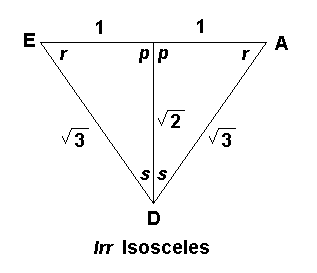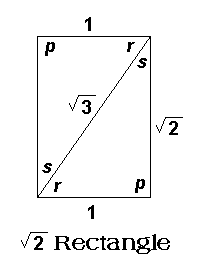Diagram 5a (left) and 5b (right)

In 5a the lrr isosceles triangle is divided by its altitude into two prs right triangles. In 5b these same two triangles share a common hypotenuse to create a sqrt2 rectangle.

Diagram 5a above illustrates another important aspect of the rich geometry of the tetrahedron cross-section. When the half-base is taken as 1 unit, the altitude of the triangle is sqrt2 (= 1.414) and the sides are sqrt3 = (1.732). This geometry is what establishes the relationship between the tetrahedron cross-section and the sqrt2 rectangle. In the sqrt2 rectangle, the short side = 1, the long side = sqrt2, and the diagonal = sqrt3. Thus all that is needed to produce the sqrt2 rectangle is to place the two prs right triangles found in the cross-section so that they share a common hypotenuse, as shown in Diagram 5b.

### 5. Rich Geometric Properties of the Sqrt2 Rectangle

Investigation of this figure shows that it is geometrically unique. Besides its relation to the tetrahedron cross-section shown above, It is the only rectangular figure which, when divided in half by a line perpendicular to its long side, yields smaller equal rectangles having the same proportions as the larger figure. The only other plane geometrical figure with this property is the 45 degree right triangle.

Because of this unique property, when the rectangle is divided in half first horizontally then vertically, four smaller rectangles with the same proportions are created. Since this process could go on indefinitely with the same proportions being maintained in smaller and smaller segments, the sqrt2 rectangle appears to have what one might call a holographic quality, which easily lends itself to symbolic interpretation. For example, the process of cell division, and in general the repetition of form in various natural processes, might be symbolically represented by this geometrically self-replicating figure.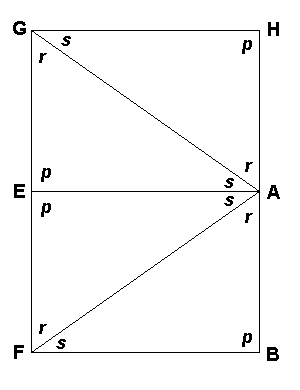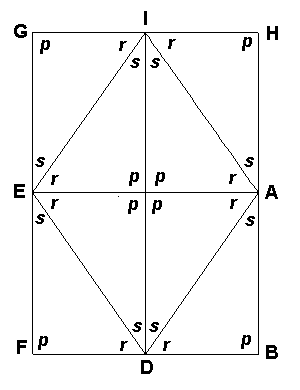Diagram 6a (left) and 6b (right)

The sqrt2 rectangle reproduces its proportions ad infinitum when bisected perpendicular to its long axis.

### 6. Representation of the Tetrahedron Cross-Section in the Sqrt2 Rectangle

When lines are drawn between all eight nodes of the rectangle, what we see are multiple repetitions of the tetrahedral cross-section. In Diagram 7a below, There are two very large cross-sections (GAF and HEB), four more 1/2 the size of the larger ones (for example EAD), and four more 1/2 that size again (with their bases at GE, EF, AB, HA). There are also multiple repetitions of prs and plt triangles. To make these stand out more clearly, Diagram 7b differentiates the various triangles using color coding. (There are many more plt triangles than just the ones shown in red. If you extend the lines of the red triangles you will see further versions of the plt triangle appearing at every other crossing line.)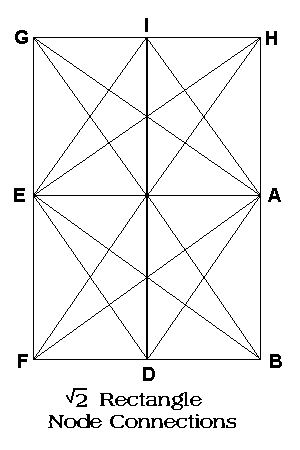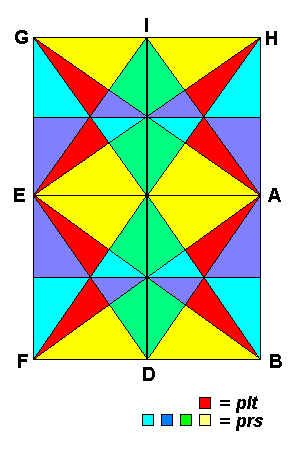Diagram 7a (left) and 7b (right)

For clarity we have left out the lines between nodes GD, IF, IB, DH. The large triangles formed between these nodes will be plt triangles.

The uniqueness of the sqrt2 rectangle shows up dramatically in Diagrams 7a-7b. The sqrt2 rectangle is truly a unique geometric figure, not only in its replicative geometry but also in its direct relationship to the tetrahedron. Because it is the only rectangle whose regular division produces smaller rectangles of the same proportions, all the triangles formed within the rectangle are repetitions of the geometry found in the two larger triangles from which the original rectangle is formed (these were prs triangles). Calculations have shown that only the sqrt2 rectangle has as many repetitions of its formative right triangle within lines drawn between the nodes -- over twice as many repetitions as in other rectangles. Furthermore, the triangles formed within the rectangle repeat the characteristic internal geometry of the tetrahedron cross-section.

This being the case, we turn our attention to the cross-section as it appears in the rectangle. Diagram 8 below shows the two interlocked cross-sections in the bottom half of the rectangle. If two tetrahedra were superimposed one on the other so that they share a common axis, their superimposed cross-sections would match this diagram (common axis is EB)

.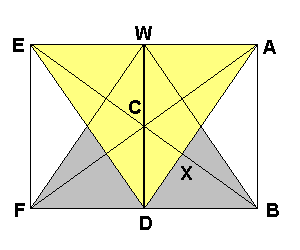Diagram 8

Tetrahedron Cross-Sections EAD and BFW within a sqrt2 rectangle.

Point C is the center of both tetrahedra. Of course, EABF is a sqrt2 rectangle (lying on its side), since it is in effect the lower 1/2 of the rectangles shown in Diagrams 7a and 7b.

With this figure we arrive at an unexpected relationship between the sqrt2 rectangle and the tetrahedral latitude. It turns out that when a circle is drawn with its center at C and its radius the length CB, this circle will represent a cross-section of a sphere enclosing the intelaced tetrahedra having cross-sections EAD and BFW. The center of the tetrahedron C is also the center of the enclosing sphere. Line EB represents the axis of both sphere and tetrahedron, and line V-V' is the equator of the sphere.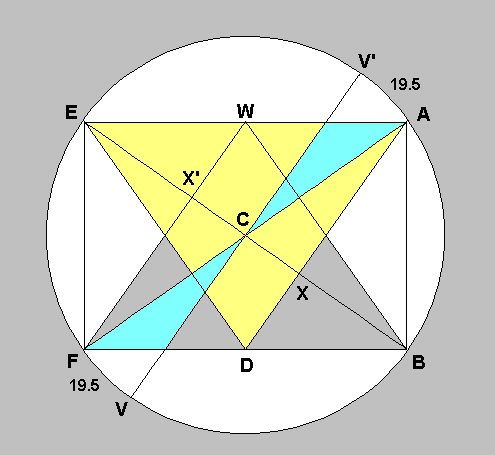Diagram 9

The sqrt2 rectangle and interlocked tetrahedron cross-sections within the cross-section of a surrounding sphere.

This analysis shows that the sqrt2 rectangle contains all the information necessary to identify the position of the 19.5 degree tetrahedral latitude and to draw an accurate cross-section of a sphere enclosing two interlocked tetrahedra. A surprising result of this analysis is that a sqrt2 rectangle inserted within a sphere so that two diagonally opposite corners of the rectangle are located at the poles, the other two corners of the rectangle will touch the sphere at the tetrahedral latitude points -- one North and one South. Thus the tetrahedral latitude can be determined by the sqrt2 rectangle without direct reference to the tetrahedron.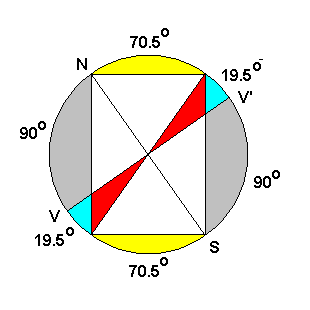Diagram 10

The sqrt2 rectangle alone defines the tetrahedral latitude on an encircling sphere. (N = North Pole, S= South Pole, V-V' = equator.)

In Diagram 10, yet another surprise: the arcs of the circle, which are defined by the corners of the sqrt2 rectangle and the equator, occupy 90, 70.5, and 19.5 degrees -- just the angles found in the plt triangle which was represented many times in the tetrahedral cross-section. This graphically demonstrates the close correspondence between the geometry of the sqrt2 rectangle and the geometry of the tetrahedron. In its internal representation of one of the five regular solids (the tetrahedron), the sqrt 2 rectangle is a geometrically "rich" figure.### 7. The Mound Pentad and the Rectangle

Finally, we come to the connection between the mound pentad and the sqrt2 rectangle. Diagram 11 shows the area of the rectangle outlined by the five mounds GABDE.As you can see, the tetrahedral cross-section is included in the pentad (EAD, axis EX).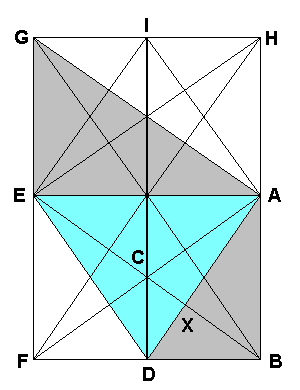Diagram 11

The area outlined by mounds GABDE within the sqrt2 rectangle.

It is true that only five of the eight nodes of the sqrt2 rectangle are represented by mounds. Certainly as far as we can detect, there are no mounds visible at the remaining three nodes. However, five mounds in the configuration shown are just the minimum necessary to make a strong geometric reference to the sqrt2 rectangle and the cross-section of the tetrahedron.

Mounds EAD form the cross-section; the line between mounds EB marks the axis; and mound G not only introduces a large prs triangle from which the rectangle may be inferred but also supplies the necessary opposite corner of the rectangle. Note that it was the presence of mound G, and the consequence that within even the tetrad of mounds GADE there are two right triangles and one isosceles, that led Dr. Crater to discover the geometry that most closely fits the mound formation. But only with the addition of the fifth mound, B, is the inference to the sqrt2 rectangle obvious (because G and B form two corners of the rectangle).

From the point of view of economy of means, the pentad configuration is a highly efficient reference to the sqrt2 rectangle and the tetrahedral geometry it contains.

### 8. Closeness of Fit

It is important to understand how closely the mound configuration actually fits the ideal pentad (which as shown above is a large portion of the sqrt2 rectangle). Dr. Crater has calculated that the average variation from the estimated mound centers is only 0.94 pixels or slightly less than 150 feet. In contrast the distances between the pentad mounds are about two miles. Furthermore the mounds are not large, being on average only about 8 x 8 pixels. Within the error margin for the mound centers the nodes of the ideal figure are on the centers of the mounds. The chart below refers to the angles between Cydonia mounds GADEB forming the pentad of mounds. Angles are in degrees. Each triangle among mounds GADEB is represented by three letters. Triangle ADE is the "lrr" tetrahedral cross-section as explained above. Note the four similar right triangles AGD, EAG, BAD, and AEB. These are "prs" right triangles.

` `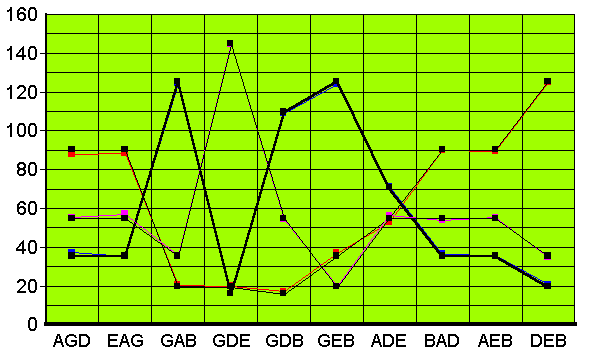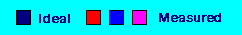` `

Since there are three angles for each triangle in the mound configuration and also in the ideal pentad, there are six lines altogether. However, the ideal and measured angles are so close that there appear to be effectively just three lines. The close overlap of the lines for the ideal angles and the lines representing the measured angles indicates the closeness of fit between the ideal and measured figures. Within measurement error, the mounds are clearly distributed according to the ideal configuration. According to Dr. Crater's calculations, the chances of this close fit occurring at random are less than one in two hundred million (based on computer simulations of random distribution patterns).

` `

### 8. Conclusion

At the outset we outlined several questions appropriate for evaluating the possibility of artificiality. One of those questions was "Is there any recognizable cultural or symbolic quality in the patterns of mounds that might provide a clue to possible meaning or utility?" There are in fact some known terrestrial cultural contexts in which the square root 2 (sqrt2) rectangle has a role. In his book The Geometry of Art and Life, Matila Ghyka discusses "Greek and Gothic Canons of Proportion" in which it is said that the proportions of certain rectangles, derivable geometrically from the square, represent harmonic balance in art and architecture.These include the sqrt2, sqrt3, and sqrt5 rectangles. They are referred to as "dynamic" rectangles. According to Ghyka, These "dynamic" rectangles were thought to produce "the most varied and satisfactory harmonic subdivisions and combinations" for use in art and architecture. In his book, he shows seven different "harmonic decompositions" of the sqrt2 rectangle, which would be used to establish a great variety of proportions perceived as aesthetically pleasing and as a basis for symbolism in art and architecture.

Among traditional classic proportions having a prominent place in design, the "golden section" or "golden ratio" (1:1.618) is perhaps much better known than the various tetrahedral proportions found in the sqrt2 rectangle. When researching the geometry of the rectangle, repeated efforts were made to determine whether the various proportions and ratios generated within the rectangle would yield, in addition, the golden section. These efforts proved unsatisfactory. However, recently I discovered a paper of Mark A. Reynolds in which a most elegant geometric solution to the problem is presented.The problem tackled by Reynolds was to discover the golden section of the circumference of a circle (what we might call the "golden arc"). Since the circumference of a circle =360 degrees, the ratio 1 to 1.618 is derived to be 137.5 to 222.5 degrees (rounded to one decimal).To construct a sqrt2 rectangle from a square, one need only start with the square and scribe the arc of a circle having as its center one corner of the square and its radius the diagonal of the square. Where the arc meets an extended side of the square determines the length of the long side of the resulting sqrt2 rectangle. This arc (having radius AC) is visible on the right in Diagram 12 below. Where the arc encounters an extension of side AD establishes point E, in the ratio of sqrt2 to side AB. Thus ABEF is a sqrt2 rectangle.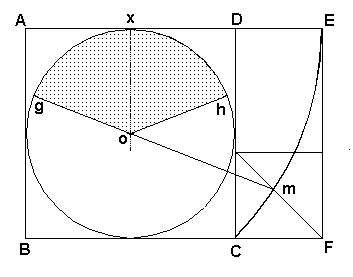Diagram 12

Mark Reynolds' derivation of the golden arc from the sqrt2 rectangle

Having drawn the arc, a circle is placed in the initial square with a diameter equal to the sides of the square. The remainder of the sqrt2 rectangle is then divided by constructing a square of sides equal to length CF (the small rectangle above this square is again a sqrt2 rectangle). Point m is now determined as the intersection of the arc with the diagonal of the small square. When a line is drawn from m through the center o of the large circle, angle gox = 68.75 degrees within a small margin of error. Doubled, this yields angle goh, the desired golden ratio arc of 137.5 degrees (the remaining arc being 222.5 degrees.) In addition to this particular derivation, Reynolds found three other pathways within the sqrt2 rectangle that yielded similar results. Thus not only are the "dynamic" architectural and aesthetic values sqrt2, sqrt3, sqrt5 present in the sqrt2 rectangle, along with the complete representation of the geometry of the tetrahedron, but also the classic golden ratio can be derived from the rectangle by simple geometric means.

One of the probable uses of such geometric measurement in the distant past lies in the possibility of laying out architectural lines having symbolically significant ratios, e.g. on the ground of a proposed site by pacing out first a square, drawing an arc on the radius of the diagonal to create a sqrt2 rectangle, and then inferring further values by utilizing the productive elements of the rectangle (such as the golden ratio as described). In a series of two articles John A. R. Legon presents data indicating that the layout of the three pyramids at Gizeh, Egypt (including the Great Pyramid) is based on a rectangle having as its sides sqrt 2 and sqrt 3, with its diagonal being sqrt 5.The values sqrt2 and sqrt3 are predominant in the sqrt2 rectangle (as well as sqrt6), and the value sqrt5 is derivable geometrically from that rectangle. From Legon's work it would appear that the layout of the Gizeh pyramids may have been influenced by the same concepts of harmonic proportion as those discussed by Ghyka.

What is most interesting about the Legon data is that it implies an application of the "dynamic rectangle" concept to the distribution of a group of architectural structures. On Mars we have, perhaps, an analogous situation. At Cydonia there is a group of formations of relatively uniform size (the mounds), distributed according to the "dynamic" sqrt 2 rectangle. The cultural implication may be that the distribution of mounds (if they are artificial) is architectural or aesthetic in intent. If so, this would be a piece of information regarding the cultural mind-set of the builders. And since the Canons of Proportion are geometrically derived -- geometry being a universal science -- there is no reason to suppose that extraterrestrial intelligence might not be responsive to the same concepts of harmonic proportion as those appreciated in the terrestrial cultural tradition.

Another possibility is that the distribution of mounds had a communicative intent, that is, it may have been intended as a kind of signal. Some critics have said that any "message" from extraterrestrials ought to be simple and easy to recognize; that any intentional pattern would have to be "obvious." But this is not necessarily the case. It could well be that a signal was created that could only be understood by a civilization advanced enough in mathematics and geometry to interpret and respond to a more complex geometric pattern. Or it could be that the "message," if that is what it is, speaks to a symbolic and aesthetic side of culture rather than a purely scientific one.

Of course, the pattern of mounds may be simply a natural formation; but if it is, it would appear to be a geological oddity because of the very low probability of the distribution having occurred by random forces. Against the idea of a natural origin, we have developed answers to our initial questions that would seem to suggest otherwise:

1.   The mound geometry conforms to a rectangular grid.
2.   The geometry is not commonplace, but unique.
3.   The geometry is not unproductive, but rich.
4.   The geometry conforms to a recognizable mode of architectural aesthetics and is capable of expressing symbolic meaning.

We conclude that the probability of either possible intentional design or radical geological anomaly for the mound configuration at Cydonia is more than sufficient to warrant high priority for continuing active investigation of this site.